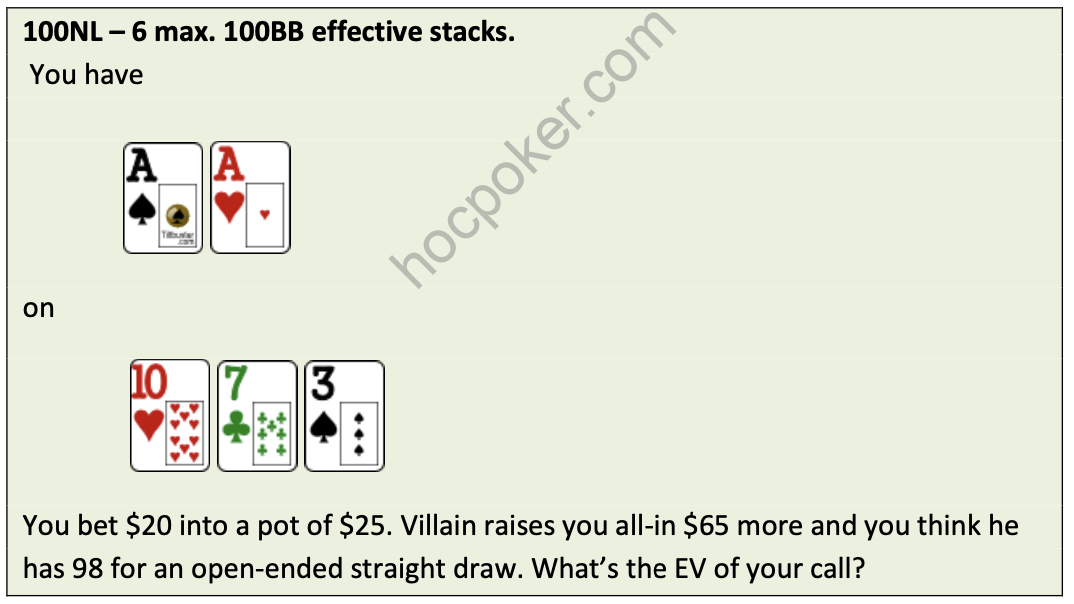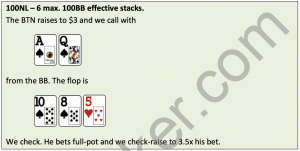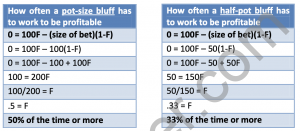# Math is Easy – Estimating Your Equity on the Flop and TurnBelow are two simple and easy methods to estimate your equity on the flop and/or turn.

They work best when you deal with small numbers of outs. Also note that Multiples of 2 are generally more accurate than Multiples of 4; however, both give very good estimates.

 Multiples of 4 – To approximate the equity you have on the flop, multiply your number of outs by 4.

o You have an open-ended draw straight on the flop. That’s 8 outs, so 8 x 4 = 32 percent to win by the river. The actual number is 31.5 percent (This is if you get to see both the turn and river cards.)

 Multiples of 2 – To approximate your equity on the turn, multiply your number of outs by 2.

o You have an open-ended draw straight on the turn. That’s 8 outs, so 8 x 2 = 16 percent to win by the river. The actual number is 17 percent.

Expected Value

Expected Value (EV) is the average amount that we stand to win or lose if we see a certain situation many times.

Let’s go over a quick hand to clarify what this means.

First, you must determine your equity against his hand. I plugged this into Poker Stove to find out that you have 65 percent equity. This means that on average, you will win 65 percent of the time and lose 35 percent of the time. So, you need to call \$65 more to win a total pot of \$110 (\$25 + \$20 + \$65). Now use the equation below to figure out your EV.

Your EV = (.65)(\$110) – (.35)(\$65) = \$71.5 – \$22.75

= \$48.75 (roughly half of a buy-in)

This means that on average, you will earn \$48.75 for every time you call his raise in this exact scenario. Thus, it is a very +EV (positive expected value) call that you should make all day because you will win a lot in the long term.

You will also lose 35 percent of the time. When you lose, you lose the \$65 you invested in the pot, but you still gain an expected value of \$48.75, or Sklansky Bucks. Just know that as long as you keep winning Sklansky Bucks, you’ll be making money when variance evens out.

So, what happens when you don’t know Villain’s exact hand? You estimate his hand range (the possible hands that he can hold).

You need to call \$68 to win \$133.50 (\$25.50 + \$20 + \$20 + \$68). To figure out the EV of your call, let’s first determine his hand range. We can safely rule out all bluffs just because it’s such a bad flop to do it on and Villain is good enough to know that.

Made hands: He can do this with AA, AK, AQ, 77, 66, A7s, A6s and 76s. We will remove some sets and two-pair hands from his range because he will often fold 77, 66, 76s, A7s, and A6s to pre-flop 3-bets. We can also take away AK some of the time because he would want to 4-bet you and get it in pre-flop.

Draws: he could have KsQs, QsJs, JsTs, Ts9s, 9s8s, 8s6s, 6s5s. Again, we will take away some of the small suited connectors because he will usually fold them pre- flop to a 3-bet. Since it’s a two-tone board, he probably wouldn’t check-raise all-in with just an open-ended straight draw.

Against this range of hands, you have 54 percent equity to win. Now let’s determine the EV of your call.

Using the equation from above:

EV = (% you win)(amount you win) – (% you lose)(amount you lose)

= (.54)(\$133.50) – (.46)(\$68) = \$72.09 – \$31.28
= \$40.81

As you can see, the EV of your call is \$30.01 (that’s 30BB at 100NL) given your read on the player’s hand ranges. You will not be able to make these kinds of calculations at the table, and that’s fine. You should do it whenever you have time. Maybe you were stuck on a hand and didn’t know if your call was correct or not. Now you can plug in the numbers to find out. Since folding is zero or neutral EV, then calling is generally correct if your EV is greater than zero.Previous post Math is Easy – Fold EquityNext post Math is Easy – Determining How Often a Bluff Has to Work to be Profitable